# sklearn.svm.LinearSVC¶

class sklearn.svm.LinearSVC(penalty='l2', loss='squared_hinge', *, dual=True, tol=0.0001, C=1.0, multi_class='ovr', fit_intercept=True, intercept_scaling=1, class_weight=None, verbose=0, random_state=None, max_iter=1000)[source]

Linear Support Vector Classification.

Similar to SVC with parameter kernel=’linear’, but implemented in terms of liblinear rather than libsvm, so it has more flexibility in the choice of penalties and loss functions and should scale better to large numbers of samples.

This class supports both dense and sparse input and the multiclass support is handled according to a one-vs-the-rest scheme.

Read more in the User Guide.

Parameters:
penalty{‘l1’, ‘l2’}, default=’l2’

Specifies the norm used in the penalization. The ‘l2’ penalty is the standard used in SVC. The ‘l1’ leads to coef_ vectors that are sparse.

loss{‘hinge’, ‘squared_hinge’}, default=’squared_hinge’

Specifies the loss function. ‘hinge’ is the standard SVM loss (used e.g. by the SVC class) while ‘squared_hinge’ is the square of the hinge loss. The combination of penalty='l1' and loss='hinge' is not supported.

dualbool, default=True

Select the algorithm to either solve the dual or primal optimization problem. Prefer dual=False when n_samples > n_features.

tolfloat, default=1e-4

Tolerance for stopping criteria.

Cfloat, default=1.0

Regularization parameter. The strength of the regularization is inversely proportional to C. Must be strictly positive.

multi_class{‘ovr’, ‘crammer_singer’}, default=’ovr’

Determines the multi-class strategy if y contains more than two classes. "ovr" trains n_classes one-vs-rest classifiers, while "crammer_singer" optimizes a joint objective over all classes. While crammer_singer is interesting from a theoretical perspective as it is consistent, it is seldom used in practice as it rarely leads to better accuracy and is more expensive to compute. If "crammer_singer" is chosen, the options loss, penalty and dual will be ignored.

fit_interceptbool, default=True

Whether to calculate the intercept for this model. If set to false, no intercept will be used in calculations (i.e. data is expected to be already centered).

intercept_scalingfloat, default=1

When self.fit_intercept is True, instance vector x becomes [x, self.intercept_scaling], i.e. a “synthetic” feature with constant value equals to intercept_scaling is appended to the instance vector. The intercept becomes intercept_scaling * synthetic feature weight Note! the synthetic feature weight is subject to l1/l2 regularization as all other features. To lessen the effect of regularization on synthetic feature weight (and therefore on the intercept) intercept_scaling has to be increased.

class_weightdict or ‘balanced’, default=None

Set the parameter C of class i to class_weight[i]*C for SVC. If not given, all classes are supposed to have weight one. The “balanced” mode uses the values of y to automatically adjust weights inversely proportional to class frequencies in the input data as n_samples / (n_classes * np.bincount(y)).

verboseint, default=0

Enable verbose output. Note that this setting takes advantage of a per-process runtime setting in liblinear that, if enabled, may not work properly in a multithreaded context.

random_stateint, RandomState instance or None, default=None

Controls the pseudo random number generation for shuffling the data for the dual coordinate descent (if dual=True). When dual=False the underlying implementation of LinearSVC is not random and random_state has no effect on the results. Pass an int for reproducible output across multiple function calls. See Glossary.

max_iterint, default=1000

The maximum number of iterations to be run.

Attributes:
coef_ndarray of shape (1, n_features) if n_classes == 2 else (n_classes, n_features)

Weights assigned to the features (coefficients in the primal problem).

coef_ is a readonly property derived from raw_coef_ that follows the internal memory layout of liblinear.

intercept_ndarray of shape (1,) if n_classes == 2 else (n_classes,)

Constants in decision function.

classes_ndarray of shape (n_classes,)

The unique classes labels.

n_features_in_int

Number of features seen during fit.

New in version 0.24.

feature_names_in_ndarray of shape (n_features_in_,)

Names of features seen during fit. Defined only when X has feature names that are all strings.

New in version 1.0.

n_iter_int

Maximum number of iterations run across all classes.

SVC

Implementation of Support Vector Machine classifier using libsvm: the kernel can be non-linear but its SMO algorithm does not scale to large number of samples as LinearSVC does. Furthermore SVC multi-class mode is implemented using one vs one scheme while LinearSVC uses one vs the rest. It is possible to implement one vs the rest with SVC by using the OneVsRestClassifier wrapper. Finally SVC can fit dense data without memory copy if the input is C-contiguous. Sparse data will still incur memory copy though.

sklearn.linear_model.SGDClassifier

SGDClassifier can optimize the same cost function as LinearSVC by adjusting the penalty and loss parameters. In addition it requires less memory, allows incremental (online) learning, and implements various loss functions and regularization regimes.

Notes

The underlying C implementation uses a random number generator to select features when fitting the model. It is thus not uncommon to have slightly different results for the same input data. If that happens, try with a smaller tol parameter.

The underlying implementation, liblinear, uses a sparse internal representation for the data that will incur a memory copy.

Predict output may not match that of standalone liblinear in certain cases. See differences from liblinear in the narrative documentation.

References

LIBLINEAR: A Library for Large Linear Classification

Examples

>>> from sklearn.svm import LinearSVC
>>> from sklearn.pipeline import make_pipeline
>>> from sklearn.preprocessing import StandardScaler
>>> from sklearn.datasets import make_classification
>>> X, y = make_classification(n_features=4, random_state=0)
>>> clf = make_pipeline(StandardScaler(),
...                     LinearSVC(random_state=0, tol=1e-5))
>>> clf.fit(X, y)
Pipeline(steps=[('standardscaler', StandardScaler()),
('linearsvc', LinearSVC(random_state=0, tol=1e-05))])

>>> print(clf.named_steps['linearsvc'].coef_)
[[0.141...   0.526... 0.679... 0.493...]]

>>> print(clf.named_steps['linearsvc'].intercept_)
[0.1693...]
>>> print(clf.predict([[0, 0, 0, 0]]))



Methods

 Predict confidence scores for samples. Convert coefficient matrix to dense array format. fit(X, y[, sample_weight]) Fit the model according to the given training data. get_params([deep]) Get parameters for this estimator. Predict class labels for samples in X. score(X, y[, sample_weight]) Return the mean accuracy on the given test data and labels. set_params(**params) Set the parameters of this estimator. Convert coefficient matrix to sparse format.
decision_function(X)[source]

Predict confidence scores for samples.

The confidence score for a sample is proportional to the signed distance of that sample to the hyperplane.

Parameters:
X{array-like, sparse matrix} of shape (n_samples, n_features)

The data matrix for which we want to get the confidence scores.

Returns:
scoresndarray of shape (n_samples,) or (n_samples, n_classes)

Confidence scores per (n_samples, n_classes) combination. In the binary case, confidence score for self.classes_ where >0 means this class would be predicted.

densify()[source]

Convert coefficient matrix to dense array format.

Converts the coef_ member (back) to a numpy.ndarray. This is the default format of coef_ and is required for fitting, so calling this method is only required on models that have previously been sparsified; otherwise, it is a no-op.

Returns:
self

Fitted estimator.

fit(X, y, sample_weight=None)[source]

Fit the model according to the given training data.

Parameters:
X{array-like, sparse matrix} of shape (n_samples, n_features)

Training vector, where n_samples is the number of samples and n_features is the number of features.

yarray-like of shape (n_samples,)

Target vector relative to X.

sample_weightarray-like of shape (n_samples,), default=None

Array of weights that are assigned to individual samples. If not provided, then each sample is given unit weight.

New in version 0.18.

Returns:
selfobject

An instance of the estimator.

get_params(deep=True)[source]

Get parameters for this estimator.

Parameters:
deepbool, default=True

If True, will return the parameters for this estimator and contained subobjects that are estimators.

Returns:
paramsdict

Parameter names mapped to their values.

predict(X)[source]

Predict class labels for samples in X.

Parameters:
X{array-like, sparse matrix} of shape (n_samples, n_features)

The data matrix for which we want to get the predictions.

Returns:
y_predndarray of shape (n_samples,)

Vector containing the class labels for each sample.

score(X, y, sample_weight=None)[source]

Return the mean accuracy on the given test data and labels.

In multi-label classification, this is the subset accuracy which is a harsh metric since you require for each sample that each label set be correctly predicted.

Parameters:
Xarray-like of shape (n_samples, n_features)

Test samples.

yarray-like of shape (n_samples,) or (n_samples, n_outputs)

True labels for X.

sample_weightarray-like of shape (n_samples,), default=None

Sample weights.

Returns:
scorefloat

Mean accuracy of self.predict(X) wrt. y.

set_params(**params)[source]

Set the parameters of this estimator.

The method works on simple estimators as well as on nested objects (such as Pipeline). The latter have parameters of the form <component>__<parameter> so that it’s possible to update each component of a nested object.

Parameters:
**paramsdict

Estimator parameters.

Returns:
selfestimator instance

Estimator instance.

sparsify()[source]

Convert coefficient matrix to sparse format.

Converts the coef_ member to a scipy.sparse matrix, which for L1-regularized models can be much more memory- and storage-efficient than the usual numpy.ndarray representation.

The intercept_ member is not converted.

Returns:
self

Fitted estimator.

Notes

For non-sparse models, i.e. when there are not many zeros in coef_, this may actually increase memory usage, so use this method with care. A rule of thumb is that the number of zero elements, which can be computed with (coef_ == 0).sum(), must be more than 50% for this to provide significant benefits.

After calling this method, further fitting with the partial_fit method (if any) will not work until you call densify.

## Examples using sklearn.svm.LinearSVC¶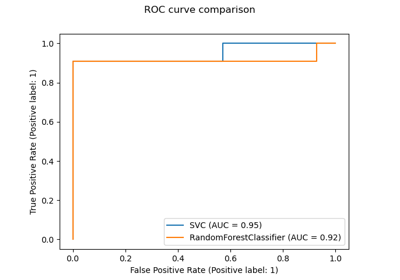Release Highlights for scikit-learn 0.22

Release Highlights for scikit-learn 0.22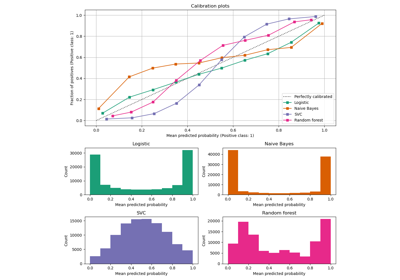Comparison of Calibration of Classifiers

Comparison of Calibration of Classifiers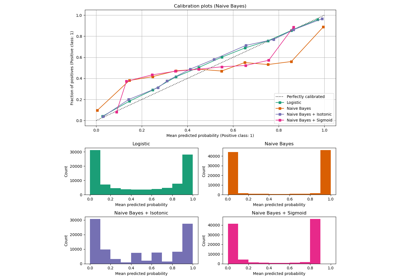Probability Calibration curves

Probability Calibration curvesPipeline ANOVA SVM

Pipeline ANOVA SVM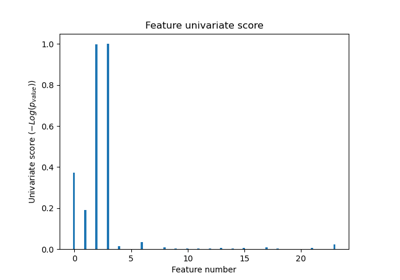Univariate Feature Selection

Univariate Feature Selection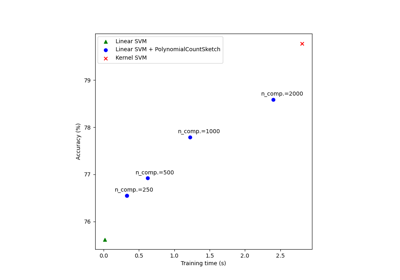Scalable learning with polynomial kernel approximation

Scalable learning with polynomial kernel approximation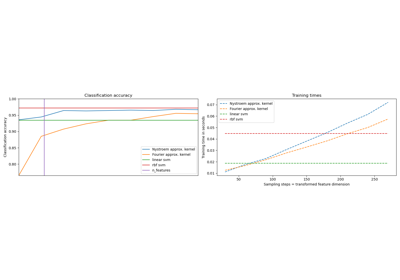Explicit feature map approximation for RBF kernels

Explicit feature map approximation for RBF kernels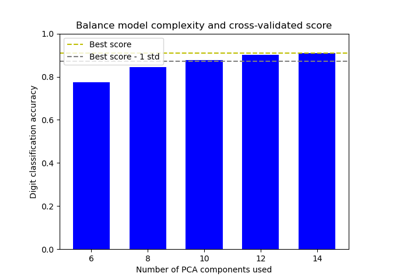Balance model complexity and cross-validated score

Balance model complexity and cross-validated score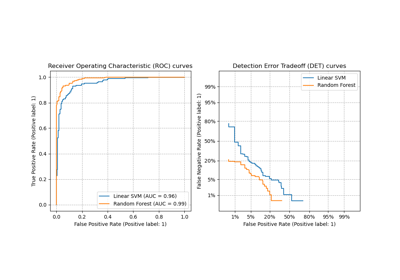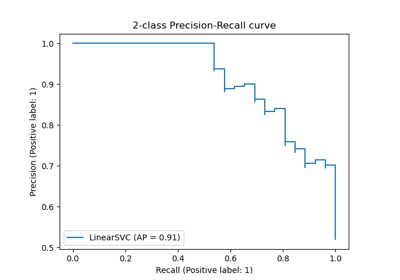Precision-Recall

Precision-RecallColumn Transformer with Heterogeneous Data Sources

Column Transformer with Heterogeneous Data Sources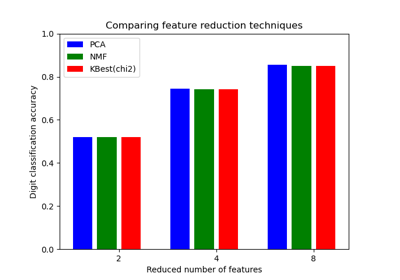Selecting dimensionality reduction with Pipeline and GridSearchCV

Selecting dimensionality reduction with Pipeline and GridSearchCV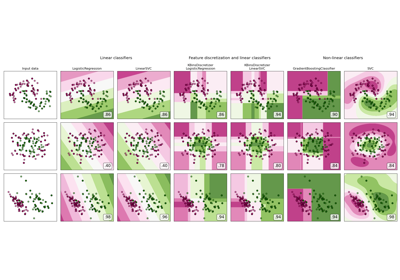Feature discretization

Feature discretization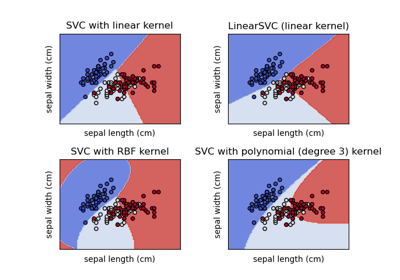Plot different SVM classifiers in the iris dataset

Plot different SVM classifiers in the iris dataset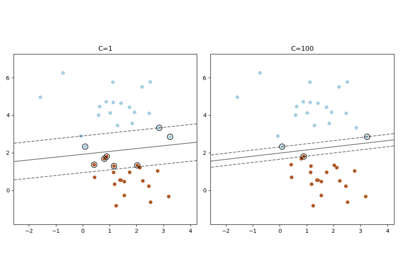Plot the support vectors in LinearSVC

Plot the support vectors in LinearSVC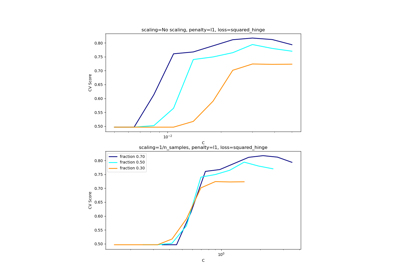Scaling the regularization parameter for SVCs

Scaling the regularization parameter for SVCsClassification of text documents using sparse features

Classification of text documents using sparse features